# 封装、继承和多态

## 封装

```class Student:
classroom = '101'

def __init__(self, name, age):
self.name = name
self.age = age

def print_age(self):
print('%s: %s' % (self.name, self.age))

# 以下是错误的用法
# 类将它内部的变量和方法封装起来，阻止外部的直接访问
print(classroom)
print_age()
```

## 继承

```class Foo(superA, superB,superC....):
class DerivedClassName(modname.BaseClassName):  ## 当父类定义在另外的模块时
```

Python支持多父类的继承机制，所以需要注意圆括号中基类的顺序，若是基类中有相同的方法名，并且在子类使用时未指定，Python会从左至右搜索基类中是否包含该方法。一旦查找到则直接调用，后面不再继续查找。

```# 父类定义
class people:

def __init__(self, name, age, weight):
self.name = name
self.age = age
self.__weight = weight

def speak(self):
print("%s 说: 我 %d 岁。" % (self.name, self.age))

# 单继承示例
class student(people):

def __init__(self, name, age, weight, grade):
# 调用父类的实例化方法
people.__init__(self, name, age, weight)

# 重写父类的speak方法
def speak(self):
print("%s 说: 我 %d 岁了，我在读 %d 年级" % (self.name, self.age, self.grade))

s = student('ken', 10, 30, 3)
s.speak()
```

### Python3的继承机制

Python3的继承机制不同于Python2。其核心原则是下面两条，请谨记！

• 子类在调用某个方法或变量的时候，首先在自己内部查找，如果没有找到，则开始根据继承机制在父类里查找。
• 根据父类定义中的顺序，以深度优先的方式逐一查找父类！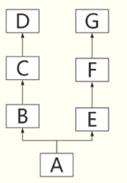```class D:
pass

class C(D):
pass

class B(C):
def show(self):
print("i am B")
pass

class G:
pass

class F(G):
pass

class E(F):
def show(self):
print("i am E")
pass

class A(B, E):
pass

a = A()
a.show()
```

```class D:
def show(self):
print("i am D")
pass

class C(D):
pass

class B(C):

pass

class G:
pass

class F(G):
pass

class E(F):
def show(self):
print("i am E")
pass

class A(B, E):
pass

a = A()
a.show()
```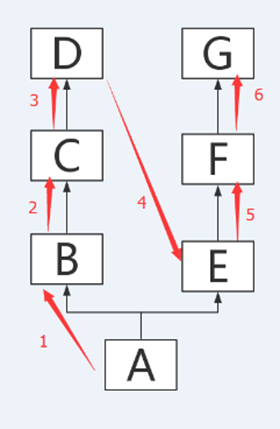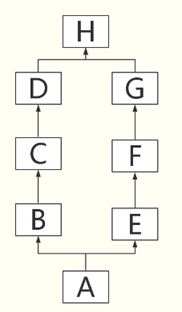```class H:
def show(self):
print("i am H")
pass

class D(H):
pass

class C(D):
pass

class B(C):
pass

class G(H):
pass

class F(G):
pass

class E(F):
def show(self):
print("i am E")
pass

class A(B, E):
pass

a = A()
a.show()
```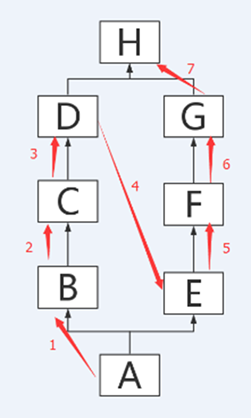```class D():
pass

class G():
def show(self):
print("i am G")
pass

class F(G):
pass

class C(D):
pass

class B(C,F):
pass

class E(F):
def show(self):
print("i am E")
pass

class A(B, E):
pass
```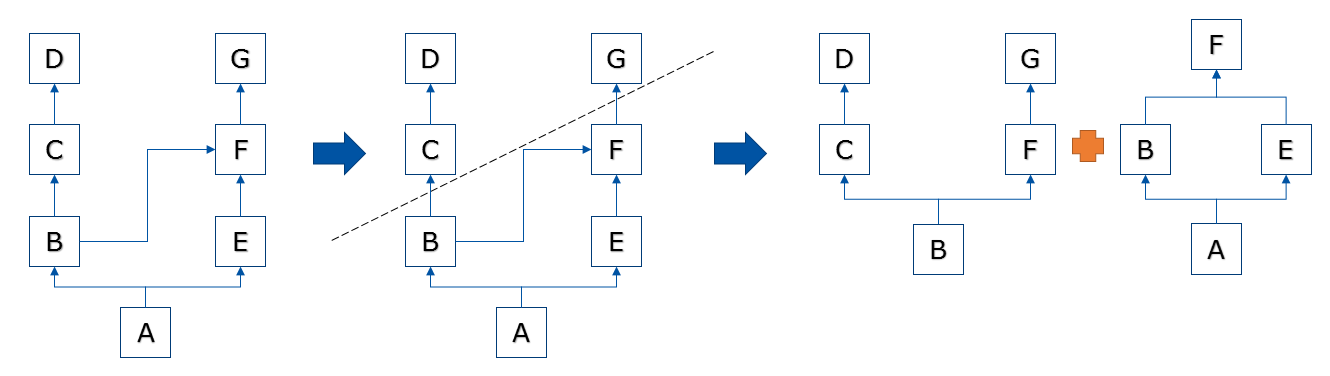### super()函数：

```class A:
def __init__(self, name):
self.name = name
print("父类的__init__方法被执行了！")
def show(self):
print("父类的show方法被执行了！")

class B(A):
def __init__(self, name, age):
super(B, self).__init__(name=name)
self.age = age

def show(self):
super(B, self).show()

obj = B("jack", 18)
obj.show()
```

## 多态

```class Animal:

def kind(self):
print("i am animal")

class Dog(Animal):

def kind(self):
print("i am a dog")

class Cat(Animal):

def kind(self):
print("i am a cat")

class Pig(Animal):

def kind(self):
print("i am a pig")

# 这个函数接收一个animal参数，并调用它的kind方法
def show_kind(animal):
animal.kind()

d = Dog()
c = Cat()
p = Pig()

show_kind(d)
show_kind(c)
show_kind(p)

------------------

i am a dog
i am a cat
i am a pig
```

```class Job:

def kind(self):
print("i am not animal, i am a job")

j = Job()
show_kind(j)
```

#### 评论总数： 3